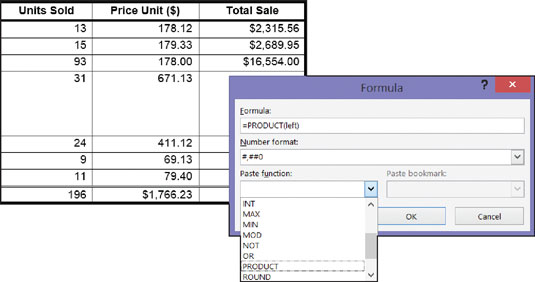##### Office 2016 All-in-One For DummiesNo, you don't have to add the figures in columns and rows yourself; Word 2016 gladly does that for you. Word can perform other mathematical calculations as well. Follow these steps to perform mathematical calculations and tell Word how to format sums and products:

1. Put the cursor in the cell that will hold the sum or product of the cells above, below, to the right, or to the left.

2. On the (Table Tools) Layout tab, click the Formula button.

Depending on the size of your screen, you may have to click the Data button first. The Formula dialog box appears, as shown. In its wisdom, Word makes an educated guess about what you want the formula to do and places a formula in the Formula box.A math formula in a table.
3. If this isn't the formula you want, delete everything except the equal sign in the Formula box, open the Paste Function drop-down list, and choose another function for the formula.

For example, choose PRODUCT to multiply figures. You may have to type left, right, above, or below in the parentheses within the formula to tell Word where it can find the figures you want to compute.

4. In the Number Format drop-down list, choose a format for your number.

5. Click OK.

Word doesn't calculate blank cells in formulas. Enter 0 in blank cells if you want them to be included in calculations. You can copy functions from one cell to another to save yourself the trouble of opening the Formula dialog box.